###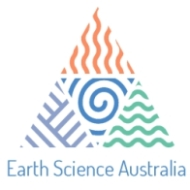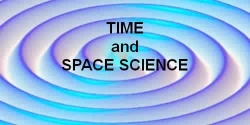#### 1.Earth Rotates About Its Axis2.Earth-Moon System Orbits a "Gravitational Centre"3.The Earth Revolves Around the Sun Once a Year4.The Sun and Earth Revolve Around the Solar System "Gravitational Centre"5. The Earth's Orbit Precesses every 25,770 years6. The Sun Revolves Around the Galaxy Once Every 250 Million Years7. The Galaxy is moving through the Universe8.Polar Variation - small but needs to be mentioned

"While most of our weather involves interactions between the earth / moon / sun / solar activity some long term weather variations may be due to lesser known earth motions"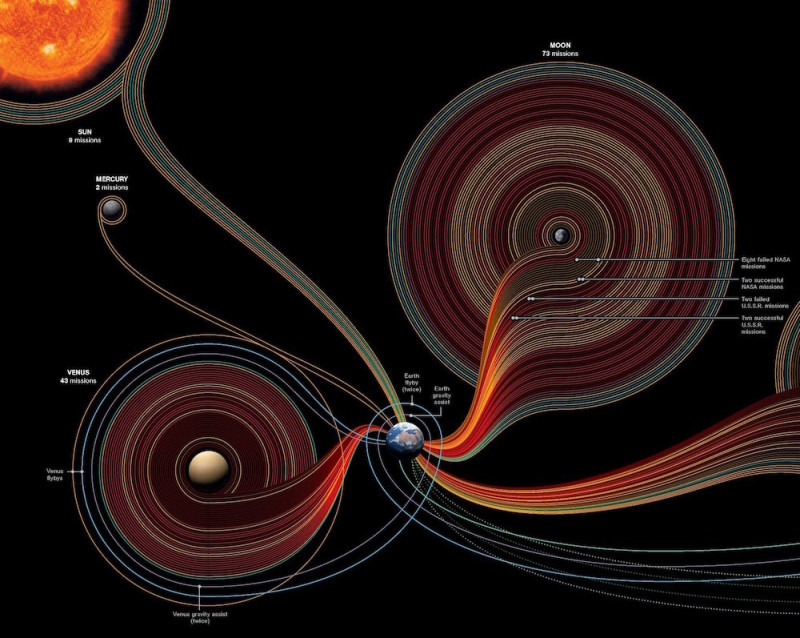Fifty years of space exploration from the earth infographic

#### 1. The Earth Rotates About Its Axis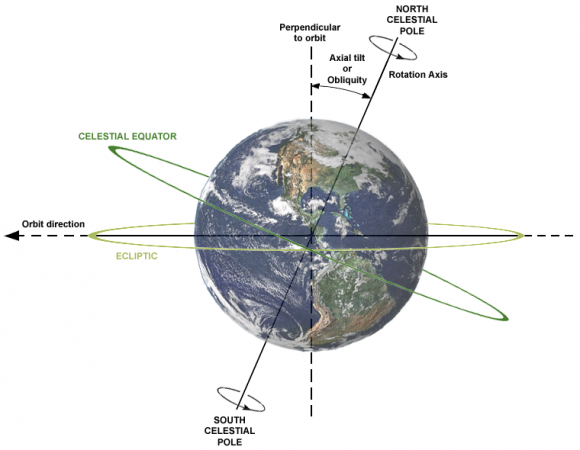• The Earth has a circumference (distance around at the Equator) of approximately 40,075 km (24,901 mi)
• The Earth rotates on its axis relative to the Sun in one "mean solar day"
• It rotates exactly 360° in one "Mean Sidereal Day" which is 86,164.0909 seconds, or 23 hrs 56 min 4.0909 seconds
• How far you travel depends on your latitude... Distance in km = 360 * (111.41288 * cos(latitude) - 0.09350 * cos(3 * latitude) + 0.00012 * cos(5 * latitude))
• speed at the equator...speed = distance/time = circumference/time = 40,075 km / 23 hrs 56 min 4.0909 seconds = 1,674 km/hr (1,040 mi/hr)
• So you are travelling faster than the speed of sound towards the eastern horizon but Earth's rotation is slowing down - about 1 second every 40,000 years.
• The North Star will be Vega instead of Polaris by the year 14,000 AD

#### 2. Earth-Moon System Orbits a "Gravitational Centre"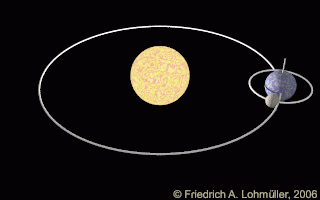• Both the Moon and the Earth orbit  a common centre of gravity
• The mass of the Earth (ME) is 5.9742 * 1024 kg, and the mass of the Moon is 7.349 * 1022 kg - the Ratio of the masses is 81.3 So the centre of gravity  is therefore located 1 unit from the Earth's centre and 81.3 units from the Moon's centre
• Earth pivots around this point once every Lunar Month

#### 3. The Earth Revolves Around the Sun Once a Year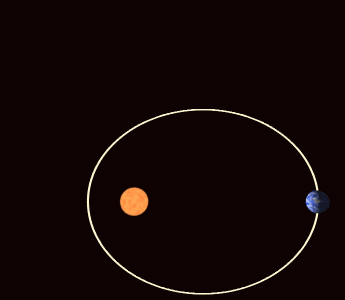• The Earth's orbit around the Sun is an ellipse  and travels at different speeds during the year - slowest at Aphelion (furthest point from the Sun) and fastest at Perihelion (closest point to the Sun)
• Earth's orbit (the path) is approximately  940,000,000 km (580,000,000 mi) in 365.2421896698 days
• So the "year" is not exactly 365¼ days (sometimes called the Julian Year).
• Earth's speed around the sun  Speed = distance/time = circumference/time = 939,951,145 km / (365.2421896698 days * 24 hr/day) = 107,229 km/hr (66,629 mi/hr)

#### 4. The Sun and Earth Revolve Around the Solar System "Gravitational Centre"

• The Sun's mass is 1.9891 * 1030 kg it is 332,950 times heavier than the Earth. The Earth does cause the Sun to wobble but it is a very tiny motion.
• On the other hand, Jupiter (the heaviest planet in the solar system) causes a much more appreciable wobble in the Sun.
• Sun wobbles around a point just outside its surface every 11.859 years (the length of Jupiter's orbit).
• Although slow, this wobble is detectable - and it also means the Earth is orbiting a slowly wobbling Sun

#### 5. The Earth's Orbit Precesses every 25,770 years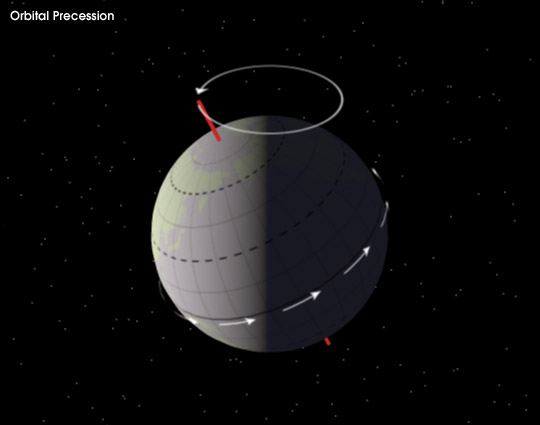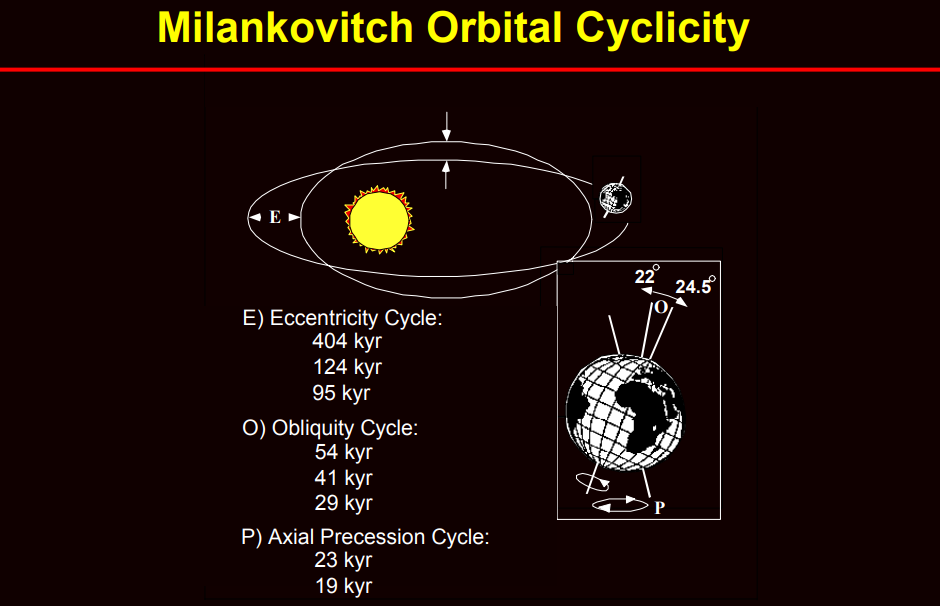• The Earth's North-South rotation axis "wobbles" like the slow rotational tilting of a spinning top over a period of 25,770 years. This is known as Precession and affects the direction in the sky to which the North Pole points and, in fact, the orientation of the entire orbital path of the Earth.
• Precession is caused by the gravitational attraction of the Sun (and the Moon) tugging on the Earth's equatorial bulge.

#### 6. The Sun Revolves Around the Galaxy Once Every 250 Million Years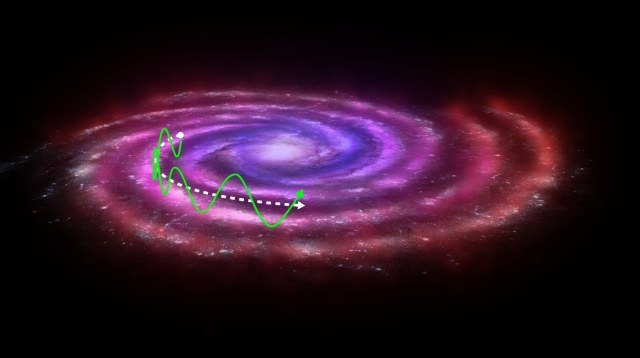• The Sun's position in the Milky Way Galaxy is about 2/3 of the way out from the center to the edge of the galactic disk, (and about 20 light years north of the galactic plane).
• Estimates of the Sun's distance from the centre of the Milky Way Galaxy range from about 25,000 to 28,000 light years (a light year is 9,460,536,000,000 km)

#### 7. The Galaxy is moving through the Universe

• The Milky Way Galaxy and the Andromeda Galaxy are approaching each other at about 400 km/s

#### 8.Polar Variation - small but needs to be mentioned

• The Earth wobbles causing the location of the North and South Poles to vary by 3 to 15 m.
• This Chandler Wobble causes the latitude of any position on the Earth to move a few metres in this 14 month cycle